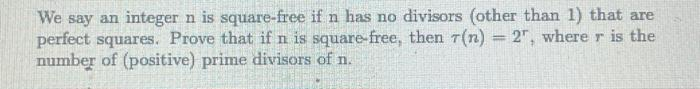# (Solved): We say an integer n is square-free if n has no divisors (other than 1 ) that are perfect squares. P ...We say an integer is square-free if has no divisors (other than 1 ) that are perfect squares. Prove that if is square-free, then , where is the number of (positive) prime divisors of .

We have an Answer from Expert